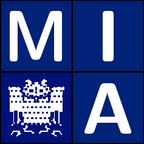Welcome to the homepage of the lecture

Convex Analysis for Visual Computing

Winter Term 2007/2008

Convex Analysis for Visual Computing

Dr. Stephan Didas

Winter Term 2007/2008

Lectures (2h) with exercises (2h); 6 ECTS points

Lectures: Monday 10–12, building E13, lecture hall 003
Tutorials: Tuesday 14–16, building E24, seminar room 3

Convexity of sets and functions is the central notion in many applications, especially in optimisation and for variational problems. In this lecture, fundamental concepts related to convexity will be explained, for example duality, sublinearity, support-function or subgradient. We always consider finite-dimensional spaces to allow for a certain simplicity and be able to cover practically relevant problems. The usefulness of those notions will be explained with example problems in visual computing.

Entrance requirementsContentsLecture NotesExercisesAssessments / ExamsReferences

Undergraduate knowledge of mathematics. For computer science students, this requirement is met by having completed the Mathematics for Computer Scientists lecture cycle.

Mathematical prerequisites which exceed the basic mathematics courses are provided within the lecture.

The course is concerned with fundamental notions of convex analysis which can be helpful for the understanding of some applications in visual computing.

Topics include:

• Convex sets and their properties
• Duality, sublinearity, support-function
• Subgradient, set-valued functions and inclusion equations

Here, you can find the hand-written notes after each lecture:

 No. Date Topic 1 29.10. Motivation and First Definitions 2 05.11. Convex Combinations and Convex Hulls 3 12.11. Theorems of Caratheodory and Helly 4 19.11. Convex Sets Attached to a Convex Set 5 26.11. Projections onto Closed Convex Sets 6 03.12. Projections onto Cones 7 04.12. Separation Results 8 10.12. Convex Functions 9 17.12. Operations Preserving Convexity of Functions 10 07.01. Legendre-Fenchel Transform and Infimal Convolution 11 14.01. Continuity Properties of Convex Functions 12 21.01. Differentiability Properties and the Subdifferential 13 28.01. Constrained Extremum Problems 14 05.02. Image Denoising with Total Variation Methods 15 11.02. Chambolle's Algorithm 16 18.02. Brouwer's Fixed Point Theorem and Applications

There is also a LaTeXed script written by Kai Hagenburg which can be found here (includes lecture 1 - 9).

 Date Exercise No. Date to be submitted 05.11. Exercise 1 12.11. 12.11. Exercise 2 19.11. 19.11. Exercise 3 26.11. 26.11. Exercise 4 03.12. 03.12. Exercise 5 10.12. 10.12. Exercise 6 17.12. 17.12. Exercise 7 07.01. 07.01. Exercise 8 14.01. 14.01. Exercise 9 21.01. 21.01. Exercise 10 28.01. 28.01. Exercise 11 05.02.

The written exam takes place on Thursday, February 28, 2008, 9 - 12 a.m., in lecture hall 003, building E1 3.
In order to qualify for the final exam, it is necessary to achieve 50% of the points of all assignment sheets in total.
It will be allowed to use the lecture notes as well as the notes taken in the tutorials during the exam.

• T. Rockafellar, Convex Analysis, Princeton University Press, 1997
• J.-B. Hiriart-Urruty and C. Lemarechal, Convex Analysis and Minimization Algorithms. Part 1: Fundamentals, Second edition, Springer, Berlin, 1996.
• M. J. Panik, Fundamentals of Convex Analysis. Kluwer, 1993.

References related to image processing include research articles and will be given in the lecture. You can find a number of books introducing the topics of the lecture in the reserved reading of the computer science library.

MIA Group